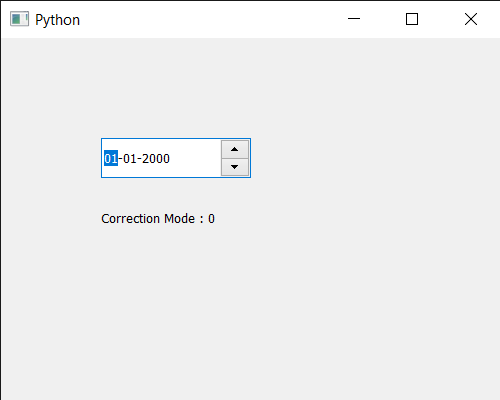Open In App

# PyQt5 QDateEdit – Getting Correction Mode

In this article we will see how we can get the correction mode to the QDateEdit. In date edit correction is very important so that user can’t enter invalid date for example if user try to enter the month value greater than 12 the current value goes back the previous valid value. In order to set the correction mode we use setCorrectionMode method, there are basically two correction modes for date edit given below

CorrectToPreviousValue : The date edit will revert to the last valid value its associated value is 0

CorrectToNearestValue : The date edit will revert to the nearest valid value, its associated value is 1 In order to do this we use correctionMode method with the QDateEdit object

Syntax : date.correctionMode()

Argument : It takes no argument

Return : It returns correction mode object but when printed it shows the value associated with it

Below is the implementation

## Python3

 `# importing libraries``from` `PyQt5.QtWidgets ``import` `*``from` `PyQt5 ``import` `QtCore, QtGui``from` `PyQt5.QtGui ``import` `*``from` `PyQt5.QtCore ``import` `*``import` `sys`  `class` `Window(QMainWindow):` `    ``def` `__init__(``self``):``        ``super``().__init__()` `        ``# setting title``        ``self``.setWindowTitle("Python ")` `        ``# setting geometry``        ``self``.setGeometry(``100``, ``100``, ``500``, ``400``)` `        ``# calling method``        ``self``.UiComponents()` `        ``# showing all the widgets``        ``self``.show()` `    ``# method for components``    ``def` `UiComponents(``self``):` `        ``# creating a QDateEdit widget``        ``date ``=` `QDateEdit(``self``)` `        ``# setting geometry of the date edit``        ``date.setGeometry(``100``, ``100``, ``150``, ``40``)` `        ``# setting correction mode``        ``date.setCorrectionMode(``0``)` `        ``# creating a label``        ``label ``=` `QLabel("GeeksforGeeks", ``self``)` `        ``# setting geometry``        ``label.setGeometry(``100``, ``150``, ``200``, ``60``)` `        ``# making label multiline``        ``label.setWordWrap(``True``)` `        ``# getting correction mode``        ``value ``=` `date.correctionMode()` `        ``# setting text to the label``        ``label.setText("Correction Mode : " ``+` `str``(value))` `# create pyqt5 app``App ``=` `QApplication(sys.argv)` `# create the instance of our Window``window ``=` `Window()` `# start the app``sys.exit(App.``exec``())`

Output :